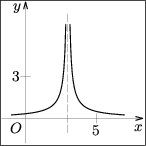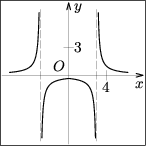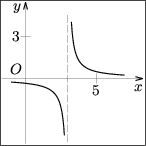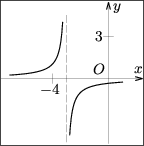CTU FCE Department of Mathematics
Thákurova 7, 166 29 Praha 6
Phone: +420 / 224 354 390

## Entrance Exam from Mathematics – online testing

There is a multiple choice of 5 answers in each of the 15 assignments of the following online test. There is always only one correct answer.

Please mark the answer you consider correct in the form on the left side.

If you click on the answer, its background should change to black. The answers can be marked in any internet browser that supports CSS pseudo class :checked. If you click on the answer and it is not marked (there is no black background), please try another internet browser. Even if the answers are not visually marked after you click on them, they are selected and will be processed when you send the test back for evaluation.

Time allotted for test is 70 minutes. When the time is up, the test is terminated and evaluated. You can finish the test before the end of trial period. Remaining time is shown in the right top hand corner of the page.

You need to have javascript enabled.

 a b c d e
1 point
 1. The line ${2x+by+1=0}$ and the line $AB$, where $A[-3,\,c]$, $B[2,\,-1]$, are identical if and only if a) $b=0\lland c=-6$ b) $b=5\lland c=1$ c) $b=5\lland c=-1$ d) $b=-5\lland c=-3$ e) $b=-3\lland c=-1$
 a b c d e
2 points
 2. An equation of the tangent line to the ellipse ${9x^2+54x+16y^2-63=0}$ at the point $[1,\,0]$ is a) ${y=0}$ b) ${x-y-1=0}$ c) ${x-1=0}$ d) ${x+y-1=0}$ e) ${y-1=0}$
 a b c d e
1 point
 3. The sum of the first three numbers of the sequence $(a_n)_{n=1}^{\infty}$, that is given by the recurrent formula ${a_{n+1}=10a_n-n}$ and by the term ${a_1=10}$, is a) $988$ b) $1\,097$ c) $1\,087$ d) $100$ e) $998$
 a b c d e
1 point
 4. If ${\cotg\alpha=1}$, then $\sin2\alpha$ equals to the number a) $0$ b) $\frac12$ c) $1$ d) $-1$ e) $\frac{\sqrt2}2$
 a b c d e
2 points
 5. The volume of the cube inscribed to the sphere with the radius $r$ is a) ${\dfrac{4\sqrt3}9\,r^3}$ b) ${\dfrac{2\sqrt2}9\,r^3}$ c) ${\dfrac{8\sqrt2}9\,r^3}$ d) ${\dfrac{3\sqrt3}5\,r^3}$ e) ${\dfrac{8\sqrt3}9\,r^3}$
 a b c d e
1 point
 6. Find the set of all solutions of the inequality ${\dfrac2{|3x-2|}<\dfrac14}$ with the unknown ${x\in\R}$. a) $(-4,\,\frac23)\cup(\frac23,\,4)$ b) $(-\infty,\,-\frac83)\cup(\frac83,\,\infty)$ c) $(-\infty,\,-2)\cup(\frac{10}3,\,\infty)$ d) $(\frac23,\,\infty)$ e) $(-2,\,\frac23)\cup(\frac23,\,\frac{10}3)$
 a b c d e
1 point
 7. The complex conjugate of ${z=(1+2\i)(5-3\i)-5+2\i}$ is a) $-6+9\i$ b) $-6-9\i$ c) $9-6\i$ d) $6-9\i$ e) $6+9\i$
 a b c d e
1 point
 8. The expression ${\dfrac{a^2+b^2}{a^2+ab}:\left(\dfrac a{a-b}-\dfrac b{a+b}\right)}$ equals to a) $\dfrac{a-b}{a+b}$, if ${a\ne0\lland a\ne b\lland a\ne-b}$ b) $\dfrac{a+b}{a-b}$, if ${a\ne0\lland a\ne b\lland a\ne-b}$ c) $\dfrac{a+b}a$, if ${a\ne0}$ d) $\dfrac{a-b}a$, if ${a\ne0\lland a\ne b\lland a\ne-b}$ e) $\dfrac a{a-b}$, if ${a\ne0\lland a\ne b\lland a\ne-b}$
 a b c d e
1 point
 9. The equation ${2\cos^2x-\sqrt 3\sin x-2=0}$ has in the interval $\langle 0,\,2\pi)$ a) no solution b) exactly three solutions c) exactly two solutions d) exactly one solution e) exactly four solutions
 a b c d e
1 point
 10. If ${\log_4y=1-2\log_4(x^2+1)+\frac {1}{2}\log_4(x+1)}$, then the number $y$ equals to a) $\dfrac{3+x}{4(x^2+1)}$ b) $\dfrac{\sqrt{x+1}}{2(x^2+1)}$ c) $\dfrac{4\sqrt{x+1}}{(x^2+1)^2}$ d) $\frac12(x-1)-2x^2$ e) $\dfrac{\sqrt{x+1}}{(x^2+1)^2}$
 a b c d e
1 point
 11. The graph of the function ${f(x)=\dfrac{1}{|x|-3}\,}$ is a)b)c) not in any of the figures d)e)a b c d e
1 point
 12. The maximal domain of the function ${f(x)=3^{\tfrac{x}{x^2-6x+8}}}$ is a) $\R-\{0,\,2,\,4\}$ b) $(2,\,4)\cup(4,\,\infty)$ c) $\R-\{2,\,4\}$ d) $(0,\,\infty)$ e) $(4,\,\infty)$
 a b c d e
2 points
 13. The set of all solutions of the inequality ${\left|\dfrac{2x+1}{x-3}+1\right|<1}$ with the unknown ${x\in\R}$ is a) $(\frac32,\,3)$ b) $(-\frac12,\,\frac54)$ c) $(-\infty,\,-\frac12)$ d) $(3,\,\infty)$ e) $(-\frac12,\,\frac32)$
 a b c d e
2 points
 14. An equilateral triangle $DEF$ is inscribed in the equilateral triangle $ABC$ with the side $a$ satisfying ${D\in AB}$, ${E\in BC}$, ${F\in CA}$. If the area of the triangle $DEF$ is equal to a third of the area of the triangle $ABC$, then its side is equal to a) ${\dfrac a4\,}$ b) ${\dfrac a{\sqrt6}\,}$ c) ${\dfrac a{\sqrt2}\,}$ d) ${\dfrac a2\,}$ e) ${\dfrac a{\sqrt3}\,}$
 a b c d e
2 points
 15. The equation ${x^2+(2m+4)x+m-1=0}$ (with the unknown $x$) a) has two different real roots, if and only if ${m=-1\llor m=0\llor m=1}$ b) has two different real roots, if and only if ${m=0}$ c) has two different real roots, if and only if ${m\in\langle -1,\,1\rangle }$ d) has two different real roots for all ${m\in\R}$ e) has no real root for all ${m\in\R}$